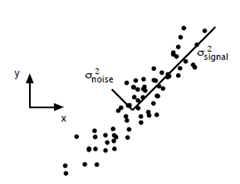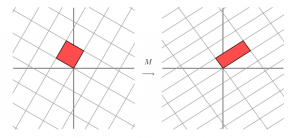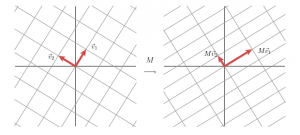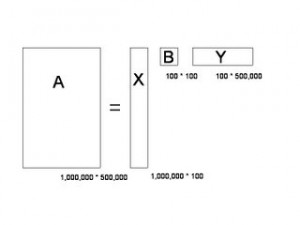# 谈谈SVD和LSA### 特征值特征向量

$M*v=\lambda*v$

v是个向量，$\lambda$是个数，那么我们称v是M的特征向量，$\lambda$是M的特征值，并且我们可以对M进行特征分解得到

$M=Q*\Lambda*Q^{-1}$

$M \approx Q_{1..k}*\Lambda_{1..k}*Q^{-1}_{1..k}$

### 奇异值分解

wiki是个好东西，你要想深入了解的话，建议还是去看wiki。奇异值分解是将矩阵变成了这样的形式

$M=U*\Sigma*V^T$$M*v_1=\sigma_1*u_1$
$M*v_2=\sigma_2*u_2$

$x=v_1*(v_1^T*x)+v_2*(v_2^T*x)$

$M*x=M*v_1*(v_1^T*x)+M*v_2*(v_2^T*x)$
$M*x=\sigma_1*u_1*(v_1^T*x)+\sigma_2*u_2*(v_2^T*x)$
$M=\sigma_1*u_1*v_1^T+\sigma_2*u_2*v_2^T$
$M=U*\Sigma*V^T$

$M*M^T=U*\Sigma*V^T*V*\Sigma^T*U^T$
$M*M^T=U*\Sigma^2*U^T$1. # -*- coding: utf-8 -*-
2.
3. from scipy import linalg, dot
4. from PIL import Image
5.
6. def main(num=5):
7.     im = Image.open('ai.jpg')
8.     pix = im.load()
9.     ma = [[], [], []]
10.     for x in xrange(im.size):
11.         for i in xrange(3):
12.             ma[i].append([])
13.         for y in xrange(im.size):
14.             for i in xrange(3):
15.                 ma[i][-1].append(pix[x, y][i])
16.     for i in xrange(3):
17.         u, s, v = linalg.svd(ma[i])
18.         u = u[:, :num]
19.         v = v[:num, :]
20.         s = s[:num]
21.         ma[i] = dot(dot(u, linalg.diagsvd(s, num, num)), v)
22.     for x in xrange(im.size):
23.         for y in xrange(im.size):
24.             ret = []
25.             for i in xrange(3):
26.                 tmp = int(ma[i][x][y])
27.                 if tmp < 0:
28.                     tmp = 0
29.                 if tmp > 255:
30.                     tmp = 255
31.                 ret.append(tmp)
32.             pix[x, y] = tuple(ret)
33.     im.show()
34.     im.save('test.jpg')
35.
36. if __name__ == '__main__':
37.     main()

### 隐含语义分析1. # -*- coding: utf-8 -*-
2.
3. import os
4. import re
5. import heapq
6. import codecs
7. from math import log
8. from scipy import linalg
9.
10. import unigram_good_turing as seg
11.
12. seg.init()
13.
14. def tfidf(docs):
15.     doclen = len(docs)+1.0
16.     for doc in docs:
17.         wordtotal = sum(doc.values())+0.0
18.         for word in doc:
19.             tf = doc[word]/wordtotal
20.             idf = log(doclen/(sum([word in tmp for tmp in docs])+1))
21.             doc[word] = tf*idf
22.     return docs
23.
24. def solve(data):
25.     re_zh, re_other = re.compile(ur"([\u4E00-\u9FA5]+)"), re.compile(ur"[^a-zA-Z0-9+#\n]")
26.     blocks = re_zh.split(data)
27.     for item in blocks:
28.         if re_zh.match(item):
29.             for i in seg.solve(item):
30.                 yield i
31.         else:
32.             tmp = re_other.split(item)
33.             for x in tmp:
34.                 if x != '':
35.                     pass
36.
37. def show(dic, p):
38.     p = heapq.nlargest(10, enumerate(p), key=lambda x:x)
39.     print ' '.join(map(lambda x:dic[x], p))
40.
41. def main():
42.     names = os.listdir('text')
43.     dic = {}
44.     cnt = 0
45.     ma = []
46.     for name in names:
47.         data = codecs.open('text/'+name, 'r', 'utf-8').read()
48.         doc = {}
49.         for word in solve(data):
50.             if not word in dic:
51.                 dic[word] = cnt
52.                 cnt += 1
53.             tmp = dic[word]
54.             if tmp not in doc:
55.                 doc[tmp] = 0
56.             doc[tmp] += 1
57.         ma.append(doc)
58.     ma = tfidf(ma)
59.     ret = []
60.     for item in ma:
61.         tmp = []
62.         for i in xrange(cnt):
63.             if i in item:
64.                 tmp.append(item[i])
65.             else:
66.                 tmp.append(0)
67.         ret.append(tmp)
68.     u, s, v = linalg.svd(ret)
69.     for i in xrange(10):
70.         show(dict(zip(dic.values(), dic.keys())), list(v[i]))
71.
72. if __name__ == '__main__':
73.     main()

### 《谈谈SVD和LSA》有 9 条评论

1.vivifox | #1

你这还真是一个程序猿的博客啊···········

•isnowfy

@vivifox: 额，那你本来以为是啥呢

2.Dreampuf | #2

够清楚，但是太单薄，多补充点各个方向的示例，将LineAlgebra的一系列方法写成个一个系列呗

•isnowfy

@Dreampuf: LineAlgebra确实是不错的题材，鉴于现在理解有限，系列以后再说吧。。。话说你的blog终于又可以访问了阿。。。

3.Alexander | #3

你好，笔误：
λ是M的特征值，不是特征向量

关于特征矩阵：特征矩阵的每个列向量都是单位向量，而且矩阵中的每个列向量互为正交。个人觉得不应该把"正交矩阵是说U∗UT=I"作为理由。这个其实更应该成为性质。

•isnowfy

@Alexander: 谢谢，特征值那个确实写错了，话说U∗UT=I这个不是正交矩阵的定义吗为什么是作为性质

4.钢板网 | #4

好文章，内容气吞山河.禁止此消息：[email protected]

5.四虎影库 | #5

四虎影库最新地址tm491.com，四虎影库最新地址tm491.com，四虎影库最新地址tm491.com